## Ordinary Differential Equation--System with Constant Coefficients

To solve the system of differential equations(1)

whereis a Matrix andand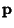are Vectors, first consider the homogeneous case with. Then the solutions to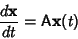(2)

are given by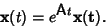(3)

But, by the Matrix Decomposition Theorem, the Matrix Exponential can be written as(4)

where the Eigenvector Matrix is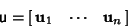(5)

and the Eigenvalue Matrix is(6)

Now consider(7)

The individual solutions are then(8)

so the homogeneous solution is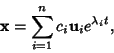(9)

where the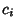s are arbitrary constants.

The general procedure is therefore

1. Find the Eigenvalues of the Matrix(, ...,) by solving the Characteristic Equation.

2. Determine the corresponding Eigenvectors, ...,.

3. Compute(10)

for, ...,. Then the Vectorswhich are Real are solutions to the homogeneous equation. Ifis a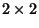matrix, the Complex vectorscorrespond to Real solutions to the homogeneous equation given by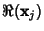and.

4. If the equation is nonhomogeneous, find the particular solution given by(11)

where the Matrixis defined by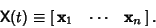(12)

If the equation is homogeneous so that, then look for a solution of the form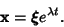(13)(14)
sois an Eigenvector andan Eigenvalue.(15)